Question

# Calculate the ΔH0 for the following reaction: NH4Cl(s) → NH3(g) + HCl(g) Given the following standard...

Calculate the ΔH0 for the following reaction: NH4Cl(s) → NH3(g) + HCl(g)

Given the following standard enthalpies of formation ΔHf0 (298 K, 1 atm) NH3(g) -46.2 kJ mol-1 ; HCl(g) -92.3 kJ mol-1 ; NH4Cl(s) -315.0 kJ mol-1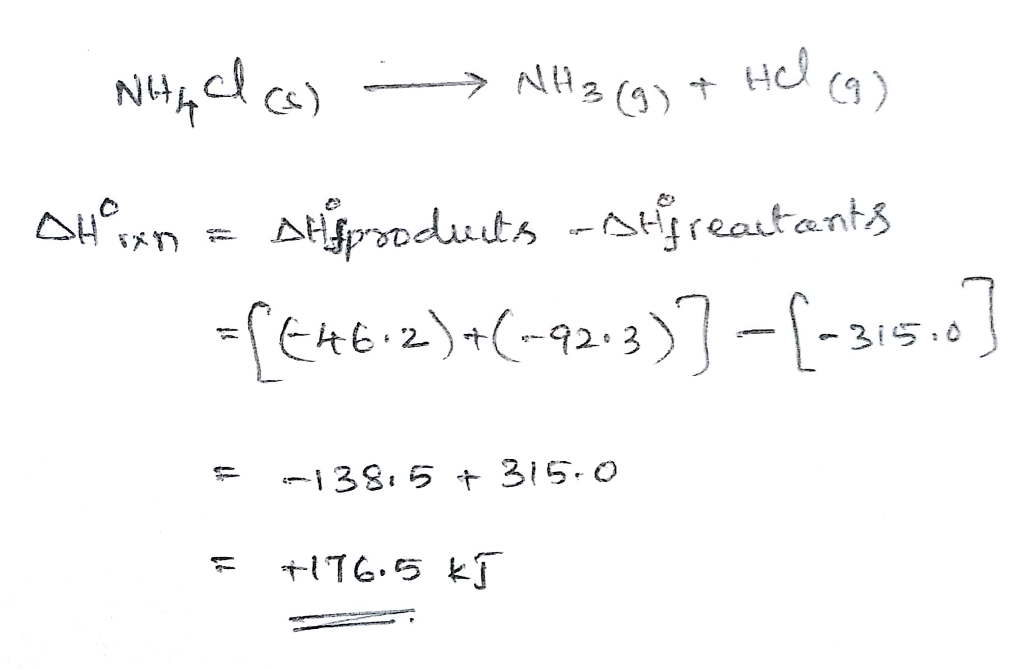#### Earn Coins

Coins can be redeemed for fabulous gifts.

Similar Homework Help Questions
• ### Consider the reaction: NH3 (g) + HCl (g) → NH4Cl (s) Given the following table of...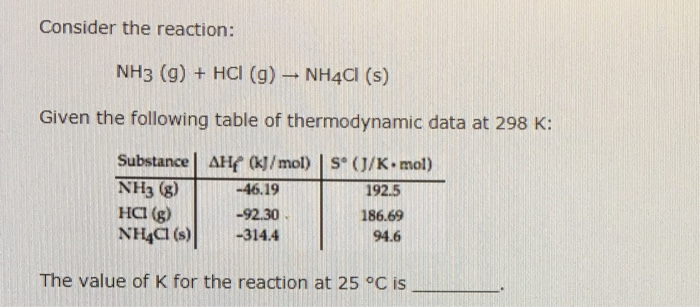Consider the reaction: NH3 (g) + HCl (g) → NH4Cl (s) Given the following table of thermodynamic data at 298 K: Substance! AH/M/mol) I s。(J/K-mol) NH3 g) HCI (g) NHCI)-3144 -46.19 -92.30 192.5 186.69 94.6 The value of K for the reaction at 25 °c is

• ### A scientist measures the standard enthaply change for the following reaction to be 80.4kJ NH4Cl(aq)=NH3(g)+HCl(aq) B...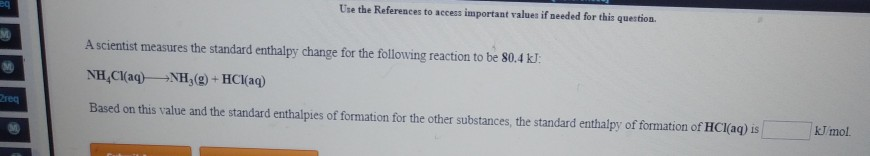A scientist measures the standard enthaply change for the following reaction to be 80.4kJ NH4Cl(aq)=NH3(g)+HCl(aq) Based on this value and the standard enthalpies of formation for the other substances, the standard enthaply of formation of HCl (aq) Use the References to access important values if needed for this question A scientist measures the standard enthalpy change for the following reaction to be 80.4 kJ. NH Cl(aq) +NH (9) + HCl(aq) G Based on this value and the standard enthalpies of...

• ### For the reaction HCl(g) + NH3(g) NH4Cl(s) AG° = -77.5 kJ and AS° = -284.8 J/K...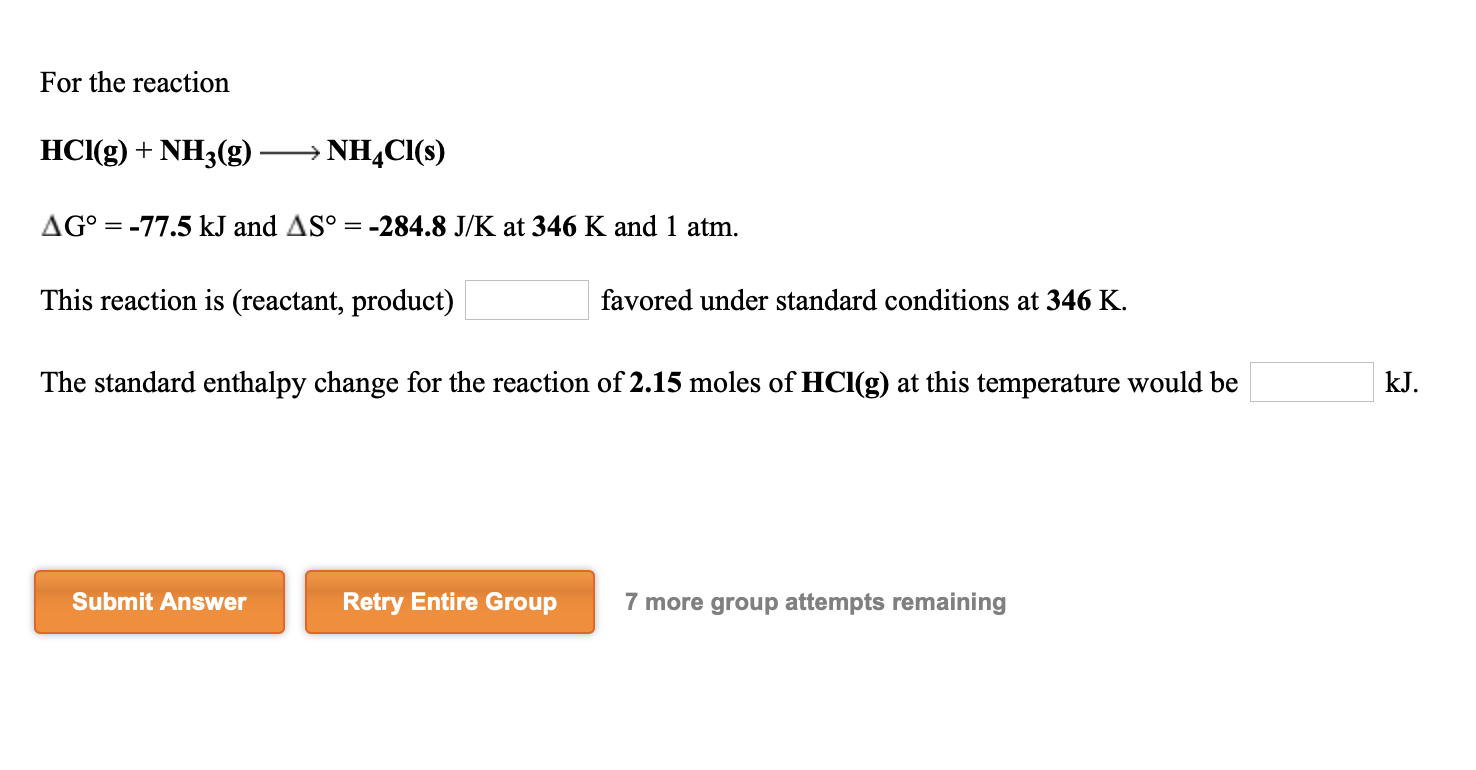For the reaction HCl(g) + NH3(g) NH4Cl(s) AG° = -77.5 kJ and AS° = -284.8 J/K at 346 K and 1 atm. This reaction is (reactant, product) favored under standard conditions at 346 K. The standard enthalpy change for the reaction of 2.15 moles of HCl(g) at this temperature would be kJ. Submit Answer Retry Entire Group 7 more group attempts remaining

• ### The equilibrium constant, Kc , for the following reaction is 5.10×10-6 at 548 K. NH4Cl(s) NH3(g)...

The equilibrium constant, Kc , for the following reaction is 5.10×10-6 at 548 K. NH4Cl(s) NH3(g) + HCl(g) If an equilibrium mixture of the three compounds in a 5.41 L container at 548 K contains 1.17 mol of NH4Cl(s) and 0.350 mol of NH3, the number of moles of HCl present is ( ) moles. The equilibrium constant, Kc , for the following reaction is 1.80×10-4 at 298 K. NH4HS(s) NH3(g) + H2S(g) If an equilibrium mixture of the three...

• ### calculate Δh0 ulate AHo (in kJ/mol) for the reaction: B2H6(g)+6 Cl2(g)2 BCls(g)+6 HCl(g) ven the following data: BCI...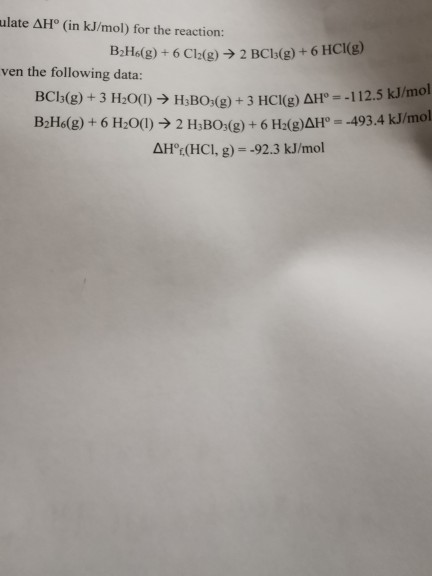calculate Δh0 ulate AHo (in kJ/mol) for the reaction: B2H6(g)+6 Cl2(g)2 BCls(g)+6 HCl(g) ven the following data: BCI5(g)+3 H2O(I) H3BO:(g)+3 HCl(g) AH° = -112.5 kJ/mol B2H&(g) + 6 H2O(1) 2 H3BO:(g) + 6 H2(g)AH°= -493.4 kJ/mol AHOC(HCI, g) -92.3 kJ/mol

• ### 1. A scientist measures the standard enthalpy change for the following reaction to be -2913.0 kJ:...

1. A scientist measures the standard enthalpy change for the following reaction to be -2913.0 kJ: 2C2H6(g) + 7 O2(g)->4CO2(g) + 6 H2O(g) Based on this value and the standard enthalpies of formation for the other substances, the standard enthalpy of formation of H2O(g) is  kJ/mol. 2. A scientist measures the standard enthalpy change for the following reaction to be -138.6 kJ : H2(g) + C2H4(g)->C2H6(g) Based on this value and the standard enthalpies of formation for the other substances, the...

• ### NH4Cl(aq) NH3(g) + HCl(aq) G° = 62.3 kJ and H° = 86.4 kJ at 305 K...

NH4Cl(aq) NH3(g) + HCl(aq) G° = 62.3 kJ and H° = 86.4 kJ at 305 K and 1 atm. This reaction is (reactant, product)----- favored under standard conditions at 305 K. The entropy change for the reaction of 2.30 moles of NH4Cl(aq) at this temperature would be ------- J/K.

• ### 7 Consider the following reaction at 298 K: NH3(g) +HCI(g)NH4CI(s) Using the thermochemical data at 298 K given below (a) Determine the AH for the reaction. (2 marks) (b) Determine the AG for the...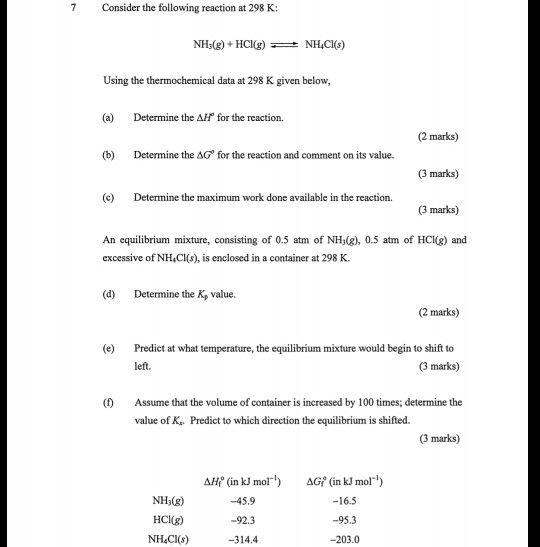7 Consider the following reaction at 298 K: NH3(g) +HCI(g)NH4CI(s) Using the thermochemical data at 298 K given below (a) Determine the AH for the reaction. (2 marks) (b) Determine the AG for the reaction and comment on its value. (3 marks) (c) Determine the maximum work done available in the reaction. (3 marks) An equilibrium mixture, consisting of 0.5 atm of NHy(), 0.5 atm of HCKg) and excessive of NH Cl(s), is enclosed in a container at 298 K...

• ### Given the information below, calculate ∆?∘?,298.15? and ∆?∘?,400? for the following reaction: ??(?) + 2???(?) →...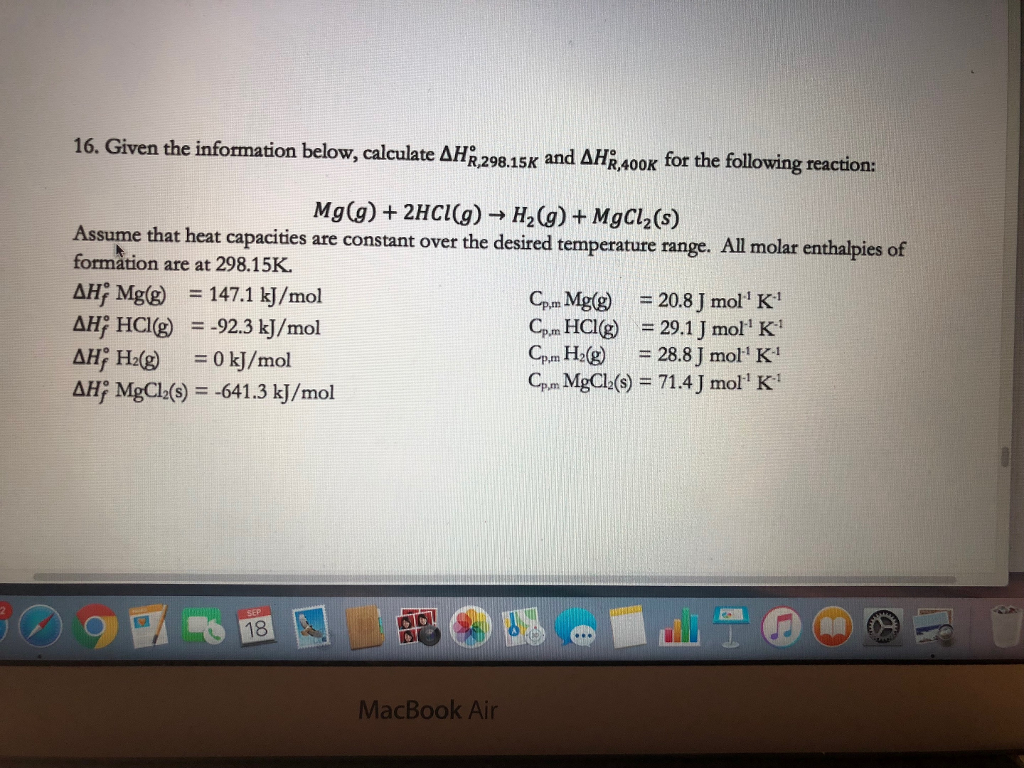Given the information below, calculate ∆?∘?,298.15? and ∆?∘?,400? for the following reaction: ??(?) + 2???(?) → ?2(?) + ????2(?) Assume that heat capacities are constant over the desired temperature range. All molar enthalpies of formation are at 298.15K. ∆?∘ Mg(g) ? = 147.1 kJ/mol ∆?∘ HCl(g) ? = -92.3 kJ/mol ∆?∘ H2(g) ? = 0 kJ/mol ∆?∘ MgCl2(s) = -641.3 kJ/mol Cp,m Mg(g) = 20.8 J mol-1 K-1 Cp,m HCl(g) = 29.1 J mol-1 K-1 Cp,m H2(g) = 28.8 J...

• ### A scientist measures the standard enthalpy change for this reaction to be - 172.0 kJ/mol: HCl(g)...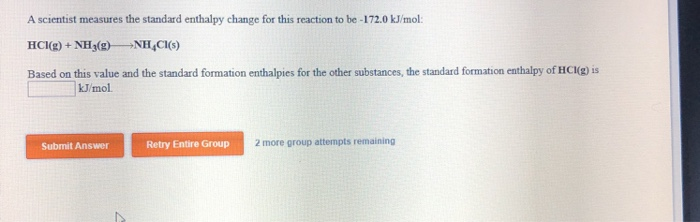A scientist measures the standard enthalpy change for this reaction to be - 172.0 kJ/mol: HCl(g) + NH3(g) →NH CH(s) Based on this value and the standard formation enthalpies for the other substances, the standard formation enthalpy of HC (g) is kJ/mol Submit Answer Retry Entire Group 2 more group attempts remaining